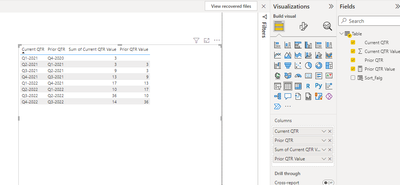cancel
Showing results for
Did you mean:Frequent Visitor

## Creating variance vs. prior period without Date Table?

Hello, due to the structure of my org's fiscal calendar, I'm unable to use a date table to calculate Quarter-over-quarter variance.

Can I more easily create a variance measure if I have a prior quarter column, which I could then use as a reference to create a "prior quarter" value column? If so, what would this look like in DAX? Envisioning it as orange below. Thanks!

 Current QTR Prior QTR Current QTR Value Prior Qtr Value? Q1-2022 Q4-2021 5 2 Q2-2022 Q1-2022 8 5 Q3-2022 Q2-2022 4 8 Q4-2022 Q3-2022 3 4
1 ACCEPTED SOLUTIONCommunity Support

Hi , @jpk564

Here are the steps you can refer to :

(1)This is my test data :(2)We can click "New Column" to create a calculated column to sort in the visual :

``````Sort_Falg = var _curent_q= CONVERT( RIGHT('Table'[Prior QTR],4) & MID('Table'[Prior QTR],2,1) ,INTEGER)
return
_curent_q``````

(3)We can create a measure :

``````Prior QTR Value = var _prior_qtr =SELECTEDVALUE('Table'[Prior QTR])
var _t= FILTER( ALLSELECTED('Table'), 'Table'[Current QTR] = _prior_qtr)
return
SUMX(_t,[Current QTR Value])``````

(4)Then we can put the filed we need on the visual ,and we can sort the [Current QTR] by the column [Sort_Falg] we create , then wen can meet your need , the result is as follows:If this method does not meet your needs, you can provide us with your special sample data and the desired output sample data in the form of tables, so that we can better help you solve the problem.

Best Regards,

Aniya Zhang

If this post helps, then please consider Accept it as the solution to help the other members find it more quickly

2 REPLIES 2Community Support

Hi , @jpk564

Here are the steps you can refer to :

(1)This is my test data :(2)We can click "New Column" to create a calculated column to sort in the visual :

``````Sort_Falg = var _curent_q= CONVERT( RIGHT('Table'[Prior QTR],4) & MID('Table'[Prior QTR],2,1) ,INTEGER)
return
_curent_q``````

(3)We can create a measure :

``````Prior QTR Value = var _prior_qtr =SELECTEDVALUE('Table'[Prior QTR])
var _t= FILTER( ALLSELECTED('Table'), 'Table'[Current QTR] = _prior_qtr)
return
SUMX(_t,[Current QTR Value])``````

(4)Then we can put the filed we need on the visual ,and we can sort the [Current QTR] by the column [Sort_Falg] we create , then wen can meet your need , the result is as follows:If this method does not meet your needs, you can provide us with your special sample data and the desired output sample data in the form of tables, so that we can better help you solve the problem.

Best Regards,

Aniya Zhang

If this post helps, then please consider Accept it as the solution to help the other members find it more quicklyFrequent Visitor

Hi Aniya - thank you very much. What would this look like as a column instead of a measure? I'm looking to have this work if only the current quarter is filtered, which, as a measure, would not show a prior quarter's value. Thank you

```Prior QTR Value = var _prior_qtr =SELECTEDVALUE('Table'[Prior QTR])
var _t= FILTER( ALLSELECTED('Table'), 'Table'[Current QTR] = _prior_qtr)
return
SUMX(_t,[Current QTR Value])```